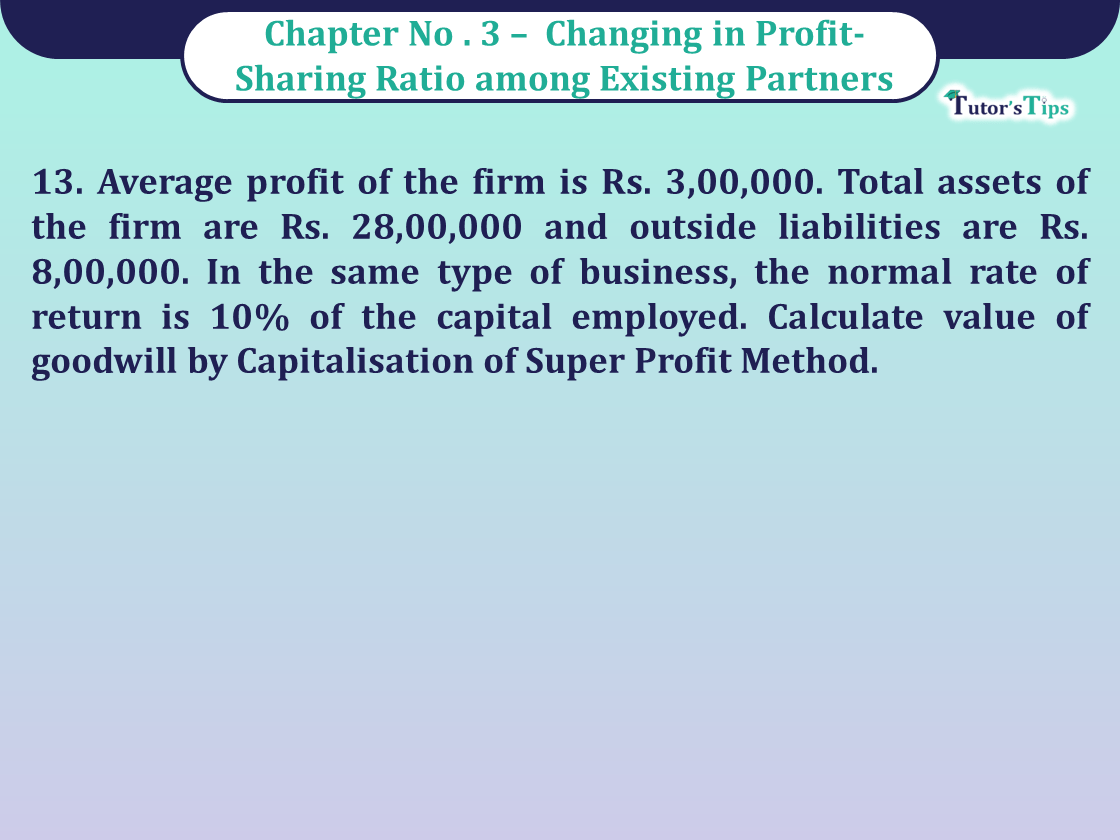# Question 13 Chapter 3 of Class 12 Part – 1 VK PublicationQuestion 13 Chapter 3 of Class 12 Part - 1 VK Publication

Question 13 Chapter 3 of Class 12 Part – 1

13. Average profit of the firm is Rs. 3,00,000. Total assets of the firm are Rs. 28,00,000 and outside liabilities are Rs. 8,00,000. In the same type of business, the normal rate of return is 10% of the capital employed. Calculate value of goodwill by Capitalisation of Super Profit Method.

## The solution of Question 13 Chapter 3 of Class 12 Part – 1: –

13. Capital Employed = Total Assets – External Liability

= 28,00,000-8,00,000 = Rs. 20,00,000

 Normal Profit = 20,00,000 X 10 100

= Rs. 2,00,000

Super Profit= Average profit- Normal Profit
= 3,00,000-2,00,000= Rs. 1,00,000

 Goodwill X Super profit X 100 Normal Rate of Return
 Goodwill X 1,00,000 X 100 10

= Rs. 10,00,000

Comment if you have any questions.

Also, Check out the solved question of previous Chapters: –